GRADE 5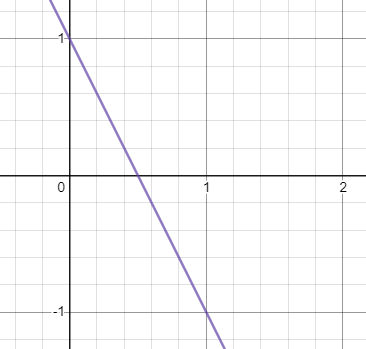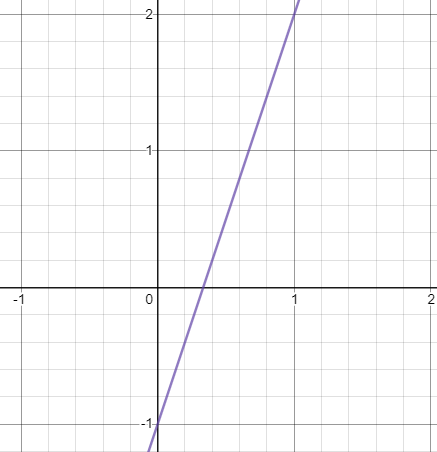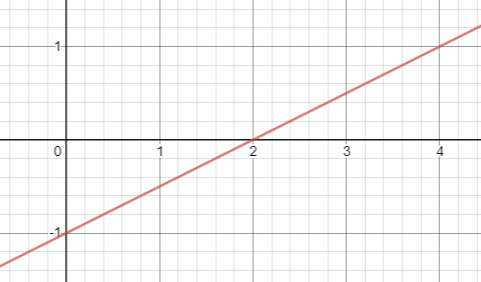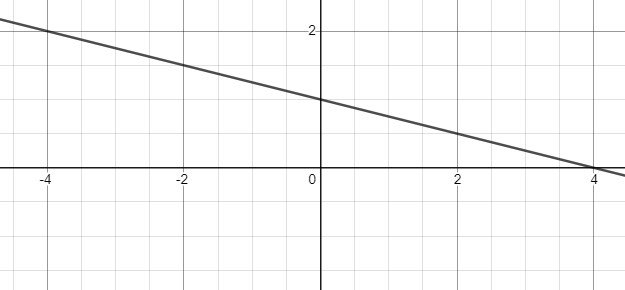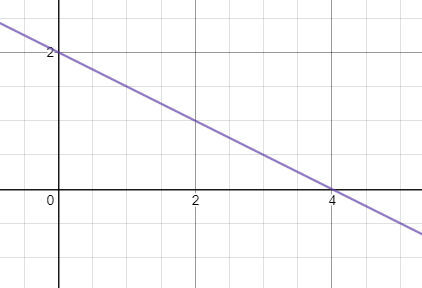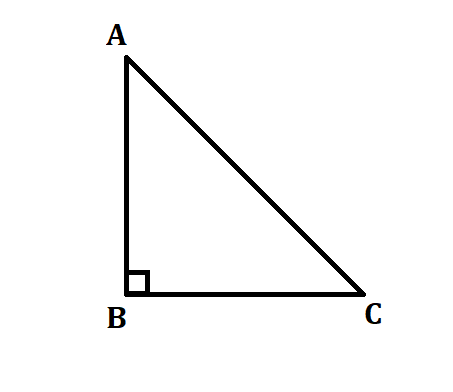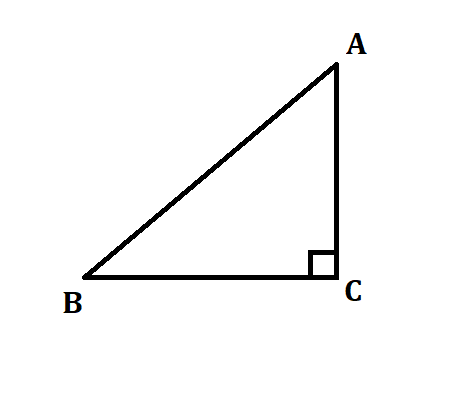a=
b=

This is a simultaneous equations question. Click to visit the maths genie
This is a column vectors question. Click to visit the maths genie
This is a question requiring Pythagoras' Theorem. Click to visit the maths genie
A question involving solving linear equations and area. You will have to set up and solve an equation to find x.
The topic is Functions, specifically Composite Functions, you will have to substitute a number in to the Composite Function.
This is a ratio problem. You need to be good at finding a missing value when at least one value is given.
Factorising quadratics - remember factorising involves putting the expression into brackets.
This is an inequalties question. Specifically about solving an inequation. It's quite similar to solving 2 equations at the same time. At the end write down the integers (whole numbers) that x could be.Click to visit the maths genie
This is a compound interest question.
The topic is reverse percentages. Don't try and work out 20% or 30% of the figure you've been given as that is not the original amount. Click to visit the maths genie
This is quite a tricky worded ratio problem. Begin by working out a ratio for the way they share the profits. Neil's share, being the smallest, would be 1. Tara's part would be double Savannah's. Then work out how many more parts of the ratio Savannah gets than Neil.
This is a question relating to straight line graphs and whether you can work out the gradient. You might know the topic as "y=mx+c" or "Straight Line Geometry".Click to visit the maths genie
Reciprocals... Every number has a reciprocal to itself. If we wanted the reciprocal of n it would be 1/n. You might have heard the word "flipped" when we talk about reciprocals
Trigonometry... You will need to know your SohCahToa and be able to find a missing angle.Click to visit the maths genie
Coming soon...
Not yet...
Not yet